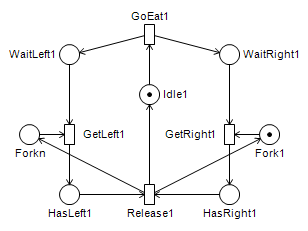# Models for the Petri Nets 2015 paper

Ákos Hajdu, András Vörös, Tamás Bartha: New search strategies for the Petri net CEGAR approach

## Distant invariant models

The reachability problem for each model is to produce a token in p0, while the marking of other places must be unchanged.

• Distant1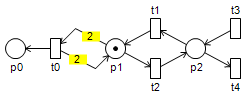This is a simple example for distant invariants. Since {t1,t2} cannot lend an extra token in p1, the distant invariant {t3,t4} is involved.

• Distant2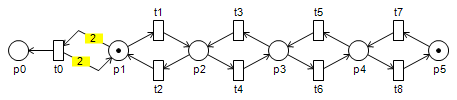In this example {t3,t4} cannot help, therefore {t5,t6} and then {t7,t8} is added.

• Distant3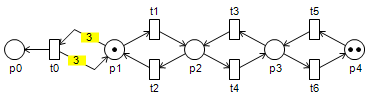This is a complex example on distant invariants. The algorithm first adds {t3,t4}, then {t5,t6}. This does not enable t0, but there is a better intermediate state, when p1 contains two tokens. Thus, {t3,t4} and {t5,t6} is added again.

• Distant4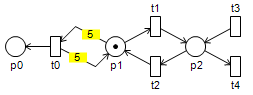This is a simple example as well. The algorithm has to add {t3,t4} five times.

• Distant5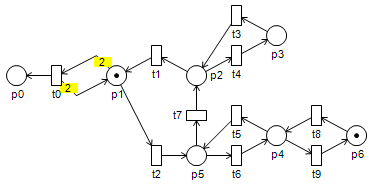In this example the T-invariant {t1,t2,t7} is lacking tokens, but there are two distant invariants: {t3,t4} and {t5,t6}. Neither of them can help, but {t5,t6} can be extended with {t8,t9}. The algorithm finds {t3,t4} first and then {t5,t6} using jump constraints.

• Distant6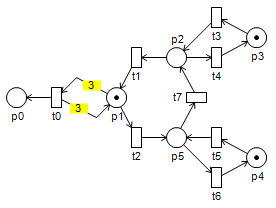There are two distant invariants for {t1,t2,t7} in this example as well, but now two tokens are required. At first the algorithm adds {t3,t4}, which does not enable t0, but there is a better intermediate state. Thus, the algortihm adds {t3,t4} again. This does not help, since {t3,t4} has only one token. Using jump constraints, the algorithm adds {t5,t6} instead of the second instance of {t3,t4}

• Distant7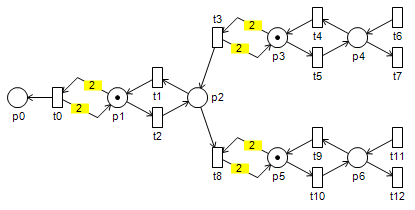This example is a recursive extension of the first example. In order to fire t3 and t4, the same problem has to be solved recursively.

## Chain model

The first number represents the number of places not connected to t0, while the second one represents the number of places connected to t0 and the number of initial tokens. Chain 2+3 can be seen in the figure below. The reachability problem is to produce a token in p0, while the marking of other places must be unchanged. This model has many T-invariants, thus a large solution space.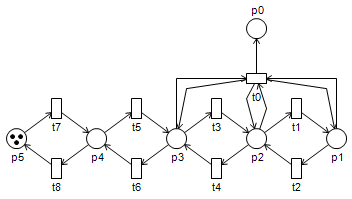## FMS model

Ciardo G.

The FMS model represents a flexible manufacturing system where different types of parts are assembled together. The parameter of this model is the number of parts to be assembled, which determines number of tokens in P1, N2 and P3. The structure of the net is fixed for each parameter. The reachability problem is to transfer the tokens from P1, N2, P3 to P12s and P3s.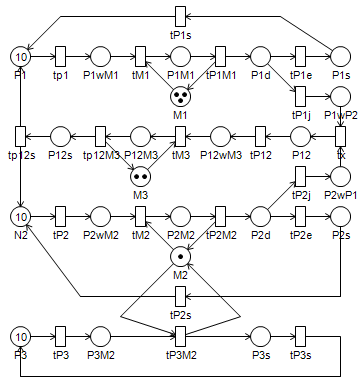## Kanban model

Ciardo G.

The Kanban model represents a production scheduling method. The parameter of this model determines the number of tokens in P1, P2, P3 and P4. The structure of the net is fixed for each parameter. The reachability problem is to transfer the tokens from P1, P2, P3, P4 to Pback1, Pback2, Pback3, Pback4.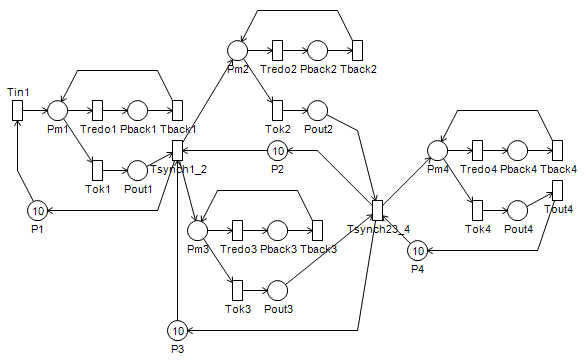## Dining philosophers model

Dijkstra E.

The Dining philosophers model is often used to illustrate the problems of parallel programming and mutual exclusion. There are n philosophers around a circular table. Each philosopher has a plate and there is a fork between each two plates. A philosopher can eat if he has a fork in both hands. Since two neighbors share a fork, at most n/2 philosophers can eat at the same time. Each philosopher is either thinking or eating. If a philosopher gets hungry, he grabs the forks next to him and eats. After eating, he puts back the forks. There is a possibility for deadlock if all the philosophers get hungry at the same time and they all grab one fork. In this case none of them can eat, therefore they will not put back the forks. The reachability problem is to reach a marking, where every second philosopher is eating. The figure below shows a part of the model, which is repeated for every philosopher. This means that the structure of the net also grows with the parameter (i.e., the number of philosophers).